# Hardy spaces

(diff) ← Older revision | Latest revision (diff) | Newer revision → (diff)

real-variable theory of, real-variable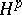theory

The real-variable Hardy spaces(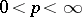) are spaces of distributions on(cf. Generalized functions, space of), originally defined as boundary values of holomorphic or harmonic functions, which have assumed an important role in modern harmonic analysis. They may be defined in terms of certain maximal functions.

Specifically, supposebelongs to the Schwartz classof rapidly decreasing smooth functions, and let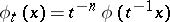for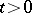. If, the space of tempered distributions, define the radial maximal function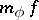and the non-tangential maximal functionby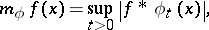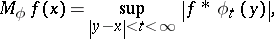wheredenotes convolution of functions. C. Fefferman and E.M. Stein [a2] (see also [a4]) proved that forand, the following conditions are equivalent (the Fefferman–Stein theorem):

1)for somewith;

2)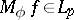for some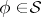with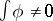;

3)for every, and in factuniformly forin a suitable bounded subset of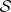.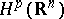is the space of all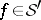that satisfy these conditions.

For,coincides with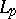, and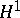is a proper subspace of. For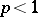,contains distributions that are not functions. A distributiononis inif and only ifis the boundary value of a harmonic functionon the upper half-plane such that, whereis the harmonic conjugate of; this is the connection with the complex-variable Hardy classes. There is a similar characterization offorin terms of systems of harmonic functions satisfying generalized Cauchy–Riemann equations; see [a2].

Another characterization offoris of great importance. A measurable functionis called a-atom (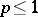) if

i)vanishes outside some ball of radius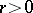and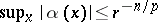;

ii)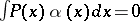for all polynomialsof degree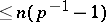. The atomic decomposition theorem (see [a4]) states that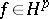if and only if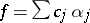, where eachis a-atom and.is a complete topological vector space for, and a Banach space for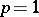, with topology defined by any of the quasi-norms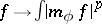,, or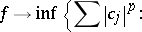By the celebrated Fefferman theorem [a2] (see also [a4]), the dual ofis the spaceof functions of bounded mean oscillation (cf. also-space). For, the dual ofis the homogeneous Lipschitz space of order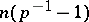; see [a3].

The spaces() andprovide an extension of the scale of-spaces (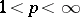) that is in many respects more natural and useful than the corresponding-spaces. Most importantly, many of the essential operations of harmonic analysis, e.g., singular integrals of Calderón–Zygmund type (cf. also Calderón–Zygmund operator; Singular integral), maximal operators and Littlewood–Paley functionals, that are well-behaved ononly forare also well-behaved onand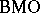. In addition, many important classes of singular distributions belong to, or are closely related to elements of, for suitable. See [a3], [a4].

The real-variabletheory can be extended to spaces other than. A rather complete extension is available in the setting of homogeneous groups, i.e., simply-connected nilpotent Lie groups (cf. also Lie group, nilpotent) with a one-parameter family of dilations; see [a3]. (These groups include, in particular,with non-isotropic dilations.) Parts of the theory have also been developed in the much more general setting of the Coifman–Weiss spaces of homogeneous type; see [a1].

How to Cite This Entry:
Hardy spaces. Encyclopedia of Mathematics. URL: http://encyclopediaofmath.org/index.php?title=Hardy_spaces&oldid=13621
This article was adapted from an original article by G.B. Folland (originator), which appeared in Encyclopedia of Mathematics - ISBN 1402006098. See original article